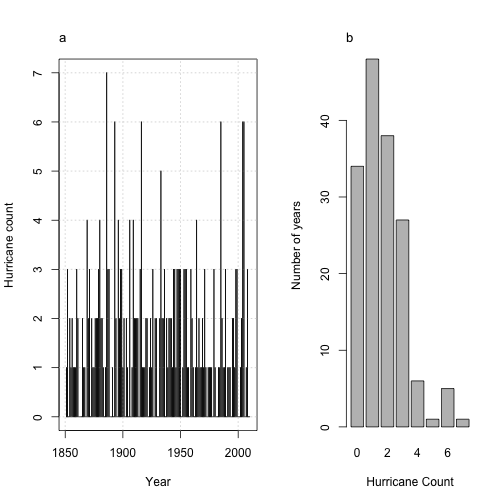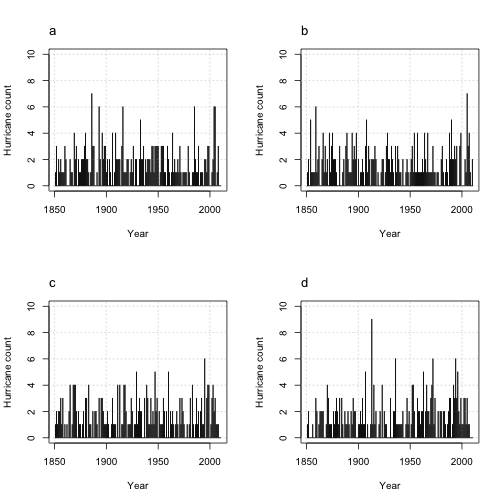# Chapter 7: Frequency Models

“Statistics is the grammar of science.”—Karl Pearson

data files: US.txt annual.RData bi.csv
packages: xtable, pscl, plotmo, earth, ggplot2, reshape
third party: roc.R

Here we focus on statistical models for understanding and predicting hurricane climate. We begin with exploratory analysis and then show you how to model counts with Poisson regression. Issues of model fit, interpretation, and prediction are considered in turn.

The topic of how to assess forecast skill is also examined including how to perform cross validation. Alternatives to the Poisson regression model are considered. Logistic regession and receiver operating characteristics are also covered.

## Counts

The data set US.txt contains a list of tropical cyclone counts by year. The counts indicate the number of hurricanes hitting in the United States (excluding Hawaii). Input the data, save them as a data frame object, and print out the first six lines by typing

con = "http://www.hurricaneclimate.com/storage/chapter-7/US.txt"

##   Year All MUS G FL E
## 1 1851   1   1 0  1 0
## 2 1852   3   1 1  2 0
## 3 1853   0   0 0  0 0
## 4 1854   2   1 1  0 1
## 5 1855   1   1 1  0 0
## 6 1856   2   1 1  1 0


The columns include year Year, number of U.S. hurricanes All, number of major U.S. hurricanes MUS, number of U.S. Gulf coast hurricanes G, number of Florida hurricanes FL, and number of East coast hurricanes E.

To make subsequent analyses easier save the number of years in the record as n and the average number hurricanes per year as rate.

n = length(H$Year) rate = mean(H$All)
n

##  160

rate

##  1.694


The average number of U.S. hurricanes is 1.69 per year over these 160 years.

Show your data. Here you plot the time series and distribution of the annual counts. Together the two plots provide a nice summary of the information in your data relevant to any modeling effort.

layout(matrix(c(1, 2), 1, 2, byrow = TRUE), widths = c(0.57, 0.43))
plot(H$Year, H$All, type = "h", xlab = "Year", ylab = "Hurricane count")
grid()
points(H$Year, H$All, type = "h")
mtext("a", side = 3, line = 1, adj = 0, cex = 1.1)
barplot(table(H$All), xlab = "Hurricane Count", ylab = "Number of years", main = "") mtext("b", side = 3, line = 1, adj = 0, cex = 1.1)The year-to-year variability and the distribution of counts appear to be consistent with a random count process. There are 34 years without a hurricane and one year (1886) with seven hurricanes. The number of years with a particular hurricane count provides a histogram. ### Poisson process The shape of the histogram suggests a Poisson distribution might be a good description for these data. The density function of the Poisson distribution shows the probability $$p$$ of obtaining a count $$x$$ when the mean count (rate) is $$\lambda$$ is given by $p(x) = \frac{\mathrm{e}^{-\lambda} \lambda^x}{x!},$ where $$\mathrm{e}$$ is the exponential function and ! is the factorial symbol. The equation indicates that probability of no events is $$p(0) = \mathrm{e}^{-\lambda}$$. With$\lambda=$1.69 hurricanes per year, the probability of no hurricanes in a random year is exp(-rate)  ##  0.1838  The probability of at least one hurricane is 1 minus this probability. Using the dpois() function you can determine the probability for any number of hurricanes. For example, to determine the probability of observing exactly one hurricane when the rate is 1.69 hurricanes per year, type dpois(x = 1, lambda = rate)  ##  0.3114  Or the probability of five hurricanes expressed in percent is dpois(5, rate) * 100  ##  2.135  Recall you can leave off the argument names in the function if the argument values are placed in the default order. The argument default order can be found by placing a question mark in front of the function name and leaving off the parentheses. This brings up the function's help page. To answer the question, 'what is the probability of two or fewer hurricanes?', you use the cumulative probability function ppois() as follows ppois(q = 2, lambda = rate)  ##  0.7589  Then to answer the question, 'what is the probability of more than two hurricanes?', you add the argument lower.tail=FALSE. ppois(q = 2, lambda = rate, lower.tail = FALSE)  ##  0.2411  ### Inhomogeneous Poisson process The Poisson distribution has the property that the variance is equal to the mean. Thus the ratio of the variance to the mean is one. You compute this ratio from your data by typing round(var(H$All)/rate, 2)

##  1.24


This says that the variance of hurricane counts is 24% larger than the mean. Is this unusual for a Poisson distribution?

You check by performing a Monte Carlo (MC) simulation experiment. A MC simulation relies on repeated random sampling. A single random sample of size $$n$$ from a Poisson distribution with a rate equal to 1.5 is obtained by typing

rpois(n = 5, lambda = 1.5)

##  2 4 3 1 2


Now repeat this $$m=1000$$ times. Let $$n$$ be the number of years in your hurricane record and $$\lambda$$ be the rate. For each sample you compute the ratio of the variance to the mean.

set.seed(3042)
ratio = numeric()
m = 1000
for (i in 1:m) {
h = rpois(n = n, lambda = rate)
ratio[i] = var(h)/mean(h)
}


The vector ratio contains 1000 values of the ratio. To help answer the 'Is this unusual?' question, you determine the proportion of ratios greater than 1.24

sum(ratio > var(H$All)/rate)/m  ##  0.028  Only 2.8% of the ratios are larger, so the answer from your MC experiment is 'yes,' the variability in hurricane counts is higher than you would expect (unusual) from a Poisson distribution with a constant rate. This indicates that the rate varies over time. Although you can compute a long-term average, some years have a higher rate than others. The variation in the rate is due to things like El Nino. So you expect more variance (extra dispersion) in counts relative to a constant rate (homogeneous Poisson) distribution. This is the basis behind seasonal forecasts. Note that a variation in the annual rate is not obvious from looking at the variation in counts. Even with a constant rate the counts will vary. You modify your MC simulation using the gamma distribution for the rate and then examine the ratio of variance to the mean from a set of Poisson counts with the variable rate. The gamma distribution describes the variability in the rate using the shape and scale parameters. The mean of the gamma distribution is the shape times the scale. You specify the shape to be 5.6 and the scale to be 0.3 so the product matches closely the long-term average count. You could, of course, choose other values that produce the same average. Now your simulation first generates 1000 random gamma values and then for each gamma 160 years of hurricane counts are generated. ratio = numeric() set.seed(3042) m = 1000 for (i in 1:m) { h = rpois(n = n, lambda = rgamma(m, shape = 5.6, scale = 0.3)) ratio[i] = var(h)/mean(h) } sum(ratio > var(H$All)/rate)/m

##  0.616


In this case you find 61.6% of the ratios are larger, so you conclude that the observed hurricane counts are more consistent with a variable rate (inhomogeneous) Poisson model.

The examples demonstrate an important use of statistics; to simulate data that have the same characteristics as your observations.

The figure below is a plot of the observed hurricane record over the 160-year period together with plots from three simulated records of the same length and having the same over-dispersed Poisson variation as the observed record. Simulated records provide a way to test hypotheses about natural variability in hurricane climate.

par(mfrow = c(2, 2))
plot(H$Year, H$All, type = "h", ylim = c(0, 10), xlab = "Year", ylab = "Hurricane count")
grid()
points(H$Year, H$All, type = "h")
mtext("a", side = 3, line = 1, adj = 0, cex = 1.1)
hh = rpois(n, lambda = rgamma(n, 5.6, scale = 0.3))
plot(H$Year, hh, type = "h", ylim = c(0, 10), xlab = "Year", ylab = "Hurricane count") grid() points(H$Year, hh, type = "h")
mtext("b", side = 3, line = 1, adj = 0, cex = 1.1)
hh = rpois(n, lambda = rgamma(n, 5.6, scale = 0.3))
plot(H$Year, hh, type = "h", ylim = c(0, 10), xlab = "Year", ylab = "Hurricane count") grid() points(H$Year, hh, type = "h")
mtext("c", side = 3, line = 1, adj = 0, cex = 1.1)
hh = rpois(n, lambda = rgamma(n, 5.6, scale = 0.3))
plot(H$Year, hh, type = "h", ylim = c(0, 10), xlab = "Year", ylab = "Hurricane count") grid() points(H$Year, hh, type = "h")
mtext("d", side = 3, line = 1, adj = 0, cex = 1.1)Summary characteristics of a 100 years of hurricanes at a coastal location may be of little value, but running a sediment transport model at that location with a large number of simulated hurricane counts will provide an assessment of the uncertainty in sediment movement caused by natural variation in hurricane frequency.